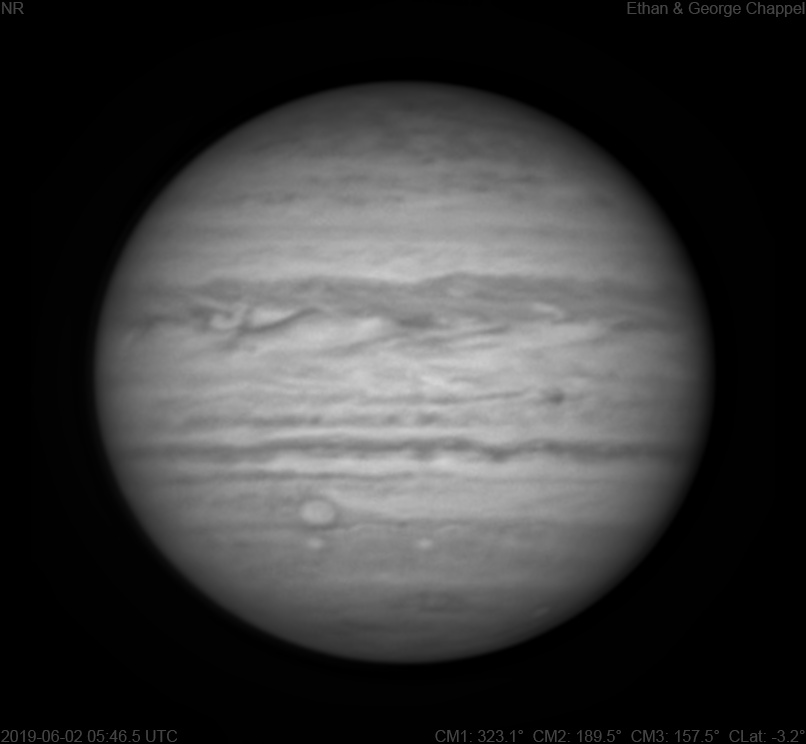# Jupiter 2019-06-02 05:46 UTC

CM1: 323.10°

CM2: 189.50°

CM3: 157.50°

CLat: -3.20°

Description

Since I needed to offload the previous RGB, a process which involves freeing a USB port for an external drive, I decided to capture some deep-red/infrared data while I had the USB hub for the focuser and filter wheel disconnected.

Notes:

• The North Temperate Belt is nothing more than a faint line.
• The North Equatorial Belt has a new rift past the central meridian.
• The Equatorial Zone contains an intense festoon approaching the CM.
• Even though the EZ has traded its typical white color for orange in RGB images, it still looks normal in infrared.
• The teal spot on the SEBn in the RGB image is very dark in infrared.
• There is a bright spot past the CM just off the SEBs.
• Oval BA is approaching the CM with the STB Ghost trailing it.
• There is a subtly dark feature setting on the South Temperate Belt. Is this a new STB structured segment?
• The South Polar Red Spot is setting.

Equipment

ZWO ASI290MM

Celestron EdgeHD 14

Logs
```FireCapture v2.6  Settings
------------------------------------
Observer=Ethan Chappel
Camera=ZWO ASI290MM
Filter=IR
Profile=Jupiter
Diameter=45.80"
Magnitude=-2.60
CMI=323.6° CMII=190.0° CMIII=158.1°  (during mid of capture)
FocalLength=12900mm (F/46)
Resolution=0.05"
Filename=2019-06-02-0547_6-EC-IR-Jup.ser
Date=2019_06_02
Start=05_46_37.696
Mid=05_47_37.705
End=05_48_37.715
Start(UT)=05_46_37.696
Mid(UT)=05_47_37.705
End(UT)=05_48_37.715
Duration=120.019s
Date_format=yyyy_MM_dd
Time_format=HH_mm_ss
LT=UT -6h
Frames captured=6293
File type=SER
Binning=no
Bit depth=8bit
Debayer=no
ROI=796x746
ROI(Offset)=0x0
FPS (avg.)=52
Shutter=19.07ms
Gain=359 (59%)
Gamma=50
Brightness=1 (off)
FPS=100 (off)
AutoGain=off
SoftwareGain=10 (off)
USBTraffic=40 (off)
AutoExposure=off
AutoHisto=75 (off)
HighSpeed=off
Histogramm(min)=0
Histogramm(max)=198
Histogramm=77%
Noise(avg.deviation)=2.65
AutoAlign=false
PreFilter=none
Limit=120 Seconds
Sensor temperature=33.0°C
Focuser position=0
FireCapture v2.6  Settings
------------------------------------
Observer=Ethan Chappel
Camera=ZWO ASI290MM
Filter=IR
Profile=Jupiter
Diameter=45.80"
Magnitude=-2.60
CMI=322.3° CMII=188.7° CMIII=156.7°  (during mid of capture)
FocalLength=14100mm (F/50)
Resolution=0.04"
Filename=2019-06-02-0545_3-EC-IR-Jup.ser
Date=2019_06_02
Start=05_44_23.913
Mid=05_45_23.917
End=05_46_23.921
Start(UT)=05_44_23.913
Mid(UT)=05_45_23.917
End(UT)=05_46_23.921
Duration=120.008s
Date_format=yyyy_MM_dd
Time_format=HH_mm_ss
LT=UT -6h
Frames captured=6293
File type=SER
Binning=no
Bit depth=8bit
Debayer=no
ROI=796x746
ROI(Offset)=0x0
FPS (avg.)=52
Shutter=19.07ms
Gain=359 (59%)
Gamma=50
Brightness=1 (off)
FPS=100 (off)
AutoGain=off
SoftwareGain=10 (off)
USBTraffic=40 (off)
AutoExposure=off
AutoHisto=75 (off)
HighSpeed=off
Histogramm(min)=0
Histogramm(max)=207
Histogramm=81%
Noise(avg.deviation)=2.69
AutoAlign=false
PreFilter=none
Limit=120 Seconds
Sensor temperature=33.0°C
Focuser position=0
```